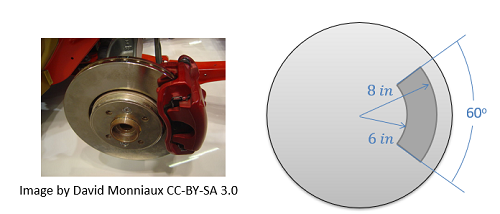﻿ Mechanics Map - Disc Friction

# Disc Friction

Disc friction is the friction that exists between the end of a rotating shaft or other rotating body and a stationary surface. Disc friction will tend to exert a moment on the bodies involved, resisting the relative rotation of the bodies. Disc friction is applicable to a wide variety of designs including end bearings, collar bearings, disc brakes, and clutches.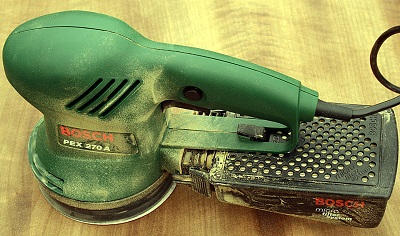This orbital sander rotates a circular sanding disc against a stationary surface. The disc friction between the sanding disc and the surface will exert a moment on both the surface and the sander. Image by Hedwig Storch CC-BY-SA 3.0

## Hollow Circular Contact Area

To start our analysis of disc friction we will use the example of a collar bearing. In this type of bearing, we will have a rotating shaft traveling through a hole in a surface. The shaft is supporting some load force as shown and a collar is used to support the shaft itself. In this case we will have a hollow circular contact area between the rotating collar and the stationary surface.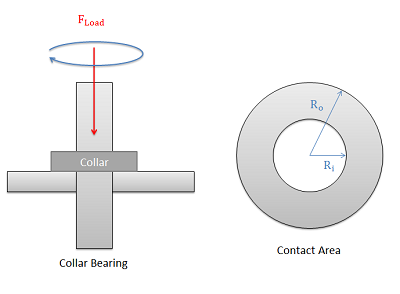In a collar bearing we will have a hollow circular contact area between the rotating collar and the stationary surface.

The friction force at any point in the contact area will be equal to the normal force at that point times the kinetic coefficient of friction at that point. If we assume a uniform pressure between the collar and the surface and a uniform coefficient of friction then we will have the same friction force exerted at all points. This does not translate however into an equal moment exerted by each point. Points further from the center of rotation will exert a larger moment than points closer to the center of rotation because they will have a larger moment arm.

 $F=\mu _k\; F_{N}$ $F_i=F_0$ $M_i\neq M_0$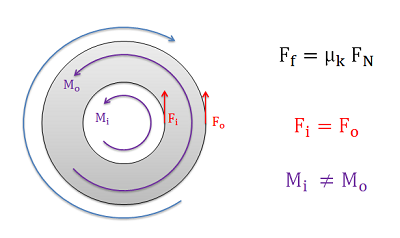Though the friction forces at any point will be the same, points along the outside surface of the contact area will exert a larger moment than points along the inside surface of the contact area.

To determine the net moment exerted by the friction forces, we will need to use calculus to sum up the individual moments over the entire contact area. The moment at each individual point will be equal to the kinetic coefficient of friction, times the normal force pressure at that point (p), times the distance from that point to the center of rotation (r).

 $M=\int_{A}dM=\int_{A}\mu _k*p*r*dA$

To simplify things we can move the constant coefficient of friction, and the constant normal force pressure term outside of the integral. We can also replace the pressure term with the load force on the bearing over the contact area. Finally, so that we can integrate over the range of R values, we can recognize that the rate of change in the area (dA) for the hollow circular areas is simply the rate of change of the r (dr) term time the circumference of the circle at r. These changes lead to the equation below.

 $M=\mu _k \left ( \frac{F_{load}}{\pi \left ( R_{0}^{2}-R_{i}^{2} \right )} \right )\int_{R_i}^{R_0}r*\left ( 2\pi r \right )dr$

Finally we can evaluate the integral from the inner radius to the outer radius. If we evaluate the integral and simplify we will end up with the final equation below.

 $M=\frac{2}{3}\mu _k\; F_{load} \left ( \frac{R_{0}^{3}-R_{i}^{3}}{R_{0}^{2}-R_{i}^{2}} \right )$

## Solid Circular Contact Area

In cases where we have a solid circular contact area such as with a solid circular shaft in an end bearing or with the orbital sander shown at the top of the page we simply set the inner radius to zero and we can simplify the formula. If we do so, the original formula is reduced to the equation below.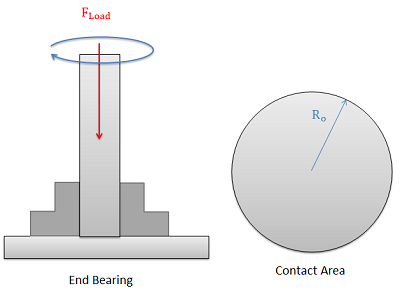An end bearing has a solid circular contact area between the rotating shaft and the stationary bearing.
 $M=\frac{2}{3}\mu _{k}F_{load}R_{0}$

## Circular Arcs (Disc Brakes)

In cases, such as in disc brakes, we may have a contact area that looks like a section of the hollow circular contact area we had earlier. For this scenario however, we have less area to exert the friction force and cause a moment, but the smaller area also causes higher pressures in that contact area for the same load force. The end result is that these terms cancel each other out and we end up with the same formula we had for the hollow circular contact area when examining a single brake pad. Most disc brakes however have a pair of pads however (one on each side of the rotating disc), so we will need to double the moment in our equation for the usual pair of pads.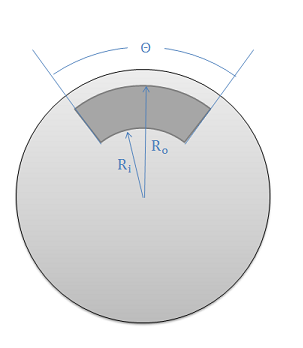The contact area in disc brakes is often approximated as a circular arc with a given contact angle (theta)
Single Brake Pad: $M=\frac{2}{3}\mu _k\; F_{load} \left ( \frac{R_{0}^{3}-R_{i}^{3}}{R_{0}^{2}-R_{i}^{2}} \right )$ $M=\frac{4}{3}\mu _k\; F_{load} \left ( \frac{R_{0}^{3}-R_{i}^{3}}{R_{0}^{2}-R_{i}^{2}} \right )$

The calculations above show that the contact angle (theta) is irrelevant to the stopping power of the brakes in theory. In practice however, larger brake pads can slightly increase the stopping power of the brakes and have other advantages such as better heat disapation.

## Worked Problems:

### Question 1:

A disc sander is pressed against a wooden surface with a force of 50N. Assuming the kinetic coefficient between the sanding pad and the wood is .6 and the diameter of the sanding disc is .2 meters, what is the torque the motor must exert to keep the disc spinning at a constant rate?Image by Hedwig Storch CC-BY-SA 3.0.

### Question 2:

In the disc brake setup shown below, a pair of brake pads is pressed into the rotor with a force of 300 lbs. If the kinetic coefficient of friction between the brake pads and the rotor is .4, find the stopping torque exerted by the brake pads.